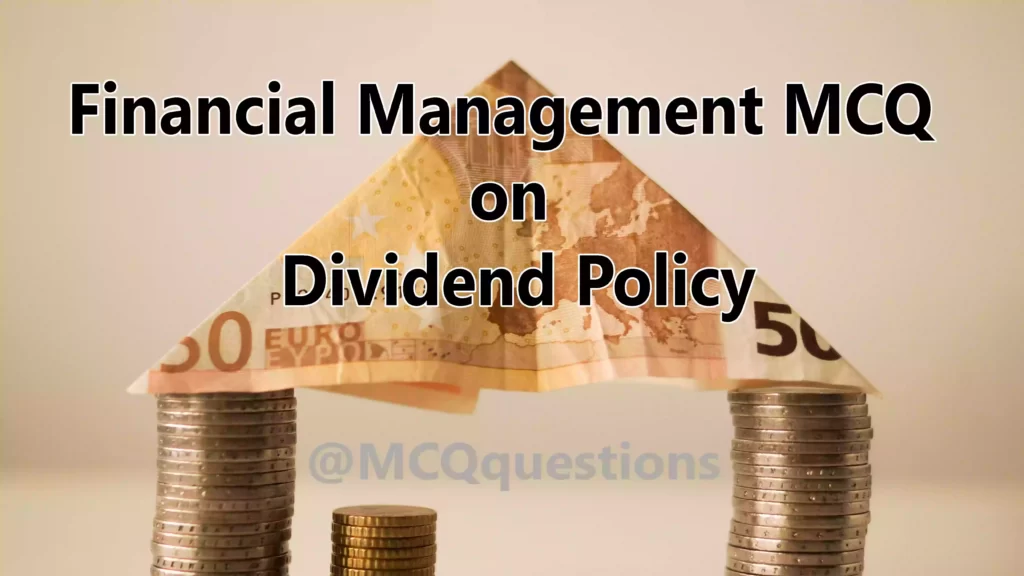# Financial Management MCQ on Dividend Policy

## MCQ on Dividend Policy

1. Dividend is defined under section ……. Under company act 2013

(a) 2(25)

(b) 2(15)

(c) 2(5)

(d) 2(35)

`Answer (d) 2(35)`

2. Retained earnings are

(a) Internal sources of funds

(b) External sources of funds

(c) Both

(d) None of the above

`Answer (a) internal sources of funds`

3. Dividend is the which component of the total rate of return

(a) Capital return

(b) Current return

(c) Both

(d) None of the above

`Answer (b) Current return`

4. Dividend can be paid in the form of

(a) Share

(b) Security

(c) Cash

(d) All of the above

`Answer (d) All of the above`

5. The Market price of the share is least affected by

(a) Steady dividend at higher level

(b) Steady dividend at lower level

(c) Steady dividend at current level

(d) None of the above

`Answer (c) Steady dividend at current level`

6. In which policy, a corporate entity follows when more profitable investment proposal are available to it and the entity needs funds?

(a) Steady dividend at higher level

(b) Steady dividend at lower level

(c) Steady dividend at current level

(d) None of the above

`Answer (b) Steady dividend at lower level`

7. The policy of a corporate entity to decide the rate of dividend in the proportion of its earnings is known as

(b) Regular dividend plus extra dividend

(c) No dividend policy

(d) Fluctuating in earning policy

`Answer (d) Fluctuating in earning policy`

8. The management of a corporate entity decides to retain a large part of profits in business and pays minimum dividend as per.

(a) Strict dividend policy

(b) Stable dividend policy

(c) Liberal dividend policy

(d) None of the above

`Answer (a) strict dividend policy`

9. Which dividend may be consider as long term dividend

(a) Liberal dividend policy

(b) Strict dividend policy

(c) Stable dividend policy

(d) None of the above

`Answer (c) Stable dividend policy`

10. A policy of consistency in the payment of dividend is known as

(a) Stable dividend policy

(b) Strict dividend policy

(c) Liberal dividend policy

(d) None of the above

`Answer (a) Stable dividend policy`

11. Which dividend ensure a fixe ratio of “dividend to income”

(a) Constant dividend per share

(b) Stable dividend payout ratio

(c) Constant dividend per share plus extra dividend

(d) None of the above

`Answer (b) Stable dividend payout ratio`

12. Dividend is derived from Latin word……..

(a) Dividendum

(b) Divend

(c) Divided

(d) None of the above

`Answer (a) Dividendum`

13. The corporate income which is given to the shareholders as a return on investment is known as

(a) Share

(b) Dividends

(c) Income

(d) Profit

`Answer (b) Dividends`

14. When the corporate declared dividend between two general meetings is known as

(a) Extra dividend

(b) Composite dividend

(c) Interim dividend

(d) None of the above

`Answer (c) Interim dividend`

15. When a company gives a part of dividend as cash and another part in scrip, bond, property is call as

(a) Extra dividend

(b) Composite dividend

(c) Interim dividend

(d) None of the above

`Answer (b) Composite dividend`

16. When a company gives dividend as share or debenture of other corporate is known as

(a) Scrip dividend

(b) Composite dividend

(c) Interim dividend

(d) None of the above

`Answer (a) Scrip dividend`

17. When a corporate pays dividend in kind rather than cash is known as

(a) Scrip dividend

(b) Composite dividend

(c) Property dividend

(d) Interim dividend

`Answer (c) Property dividend`

18. Punishment to failure to distribute dividend come under section

(a) 117

(b) 127

(c) 137

(d) 147

`Answer (b) 127`

19. Which resolution is required to be passed for the declaration of dividend in the general meeting of the company?

(a) Ordinary resolution

(b) Special resolution

(c) Both

(d) None of the above

`Answer (a) Ordinary resolution`

20. What is the rate of interest paid by company for punishment for failure to distribute dividend

(a) 8%

(b) 18%

(c) 28%

(d) 38%

`Answer (b) 18%`